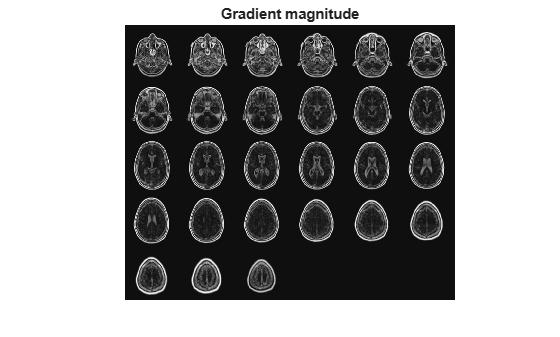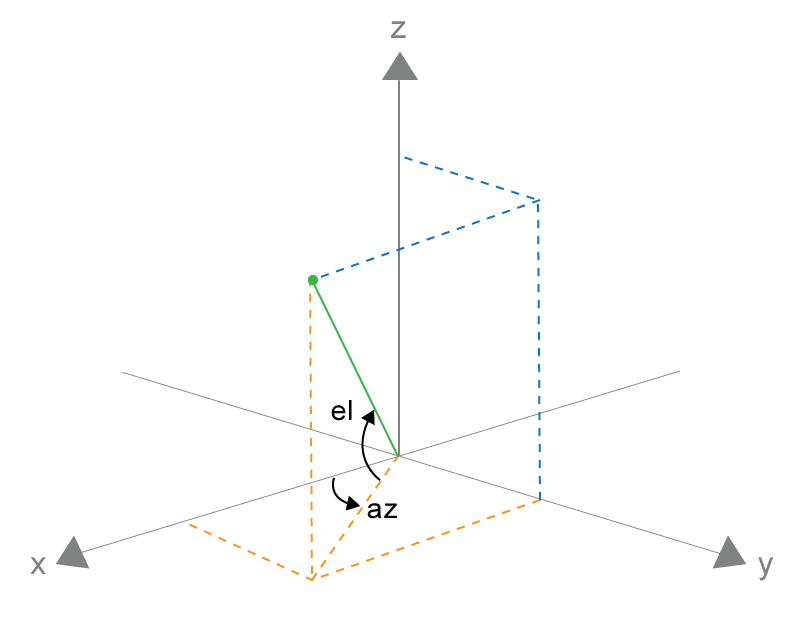Find gradient magnitude and direction of 3-D image

## Syntax

``````[Gmag,Gazimuth,Gelevation] = imgradient3(I)``````
``````[Gmag,Gazimuth,Gelevation] = imgradient3(I,method)``````
``````[Gmag,Gazimuth,Gelevation] = imgradient3(Gx,Gy,Gz)``````

## Description

example

``````[Gmag,Gazimuth,Gelevation] = imgradient3(I)``` returns the gradient magnitude, `Gmag`, gradient direction, `Gazimuth`, and gradient elevation `Gelevation` of the 3-D grayscale or binary image `I`.```
``````[Gmag,Gazimuth,Gelevation] = imgradient3(I,method)``` calculates the gradient magnitude, direction, and elevation using the specified `method`.```
``````[Gmag,Gazimuth,Gelevation] = imgradient3(Gx,Gy,Gz)``` calculates the gradient magnitude, direction, and elevation from the directional gradients `Gx`, `Gy`, and `Gz` in the x, y, and z directions, respectively.```

## Examples

collapse all

Read 3-D data into the workspace and prepare it for processing.

```volData = load('mri'); sz = volData.siz; vol = squeeze(volData.D);```

`[Gmag, Gaz, Gelev] = imgradient3(vol);`

Visualize the gradient magnitude as a montage.

```figure, montage(reshape(Gmag,sz(1),sz(2),1,sz(3)),'DisplayRange',[]) title('Gradient magnitude')```## Input Arguments

collapse all

Input image, specified as a 3-D grayscale image or 3-D binary image.

Data Types: `single` | `double` | `int8` | `int16` | `int32` | `int64` | `uint8` | `uint16` | `uint32` | `uint64` | `logical`

Gradient operator, specified as one of the following values.

Value

Meaning

`'sobel' `

Sobel gradient operator. The gradient of a pixel is a weighted sum of pixels in the 3-by-3-by-3 neighborhood. For example, in the depth (z) direction, the weights in the three planes are:

plane `z-1`plane `z`plane `z+1`
```[ 1 3 1 3 6 3 1 3 1 ] ```
```[ 0 0 0 0 0 0 0 0 0 ] ```
```[ -1 -3 -1 -3 -6 -3 -1 -3 -1 ] ```

`'prewitt'`

Prewitt gradient operator. The gradient of a pixel is a weighted sum of pixels in the 3-by-3-by-3 neighborhood. For example, in the depth (z) direction, the weights in the three planes are:

plane `z-1`plane `z`plane `z+1`
```[ 1 1 1 1 1 1 1 1 1 ] ```
```[ 0 0 0 0 0 0 0 0 0 ] ```
```[ -1 -1 -1 -1 -1 -1 -1 -1 -1 ] ```

`'central' `

Central difference gradient. The gradient of a pixel is a weighted difference of neighboring pixels. For example, in the depth (z) direction, ```dI/dz = (I(z+1) - I(z-1))/2```.

`'intermediate'`

Intermediate difference gradient. The gradient of a pixel is the difference between an adjacent pixel and the current pixel. For example, in the depth (z) direction, ```dI/dz = I(z+1) - I(z)```.

When applying the gradient operator at the boundaries of the image, `imgradient3` assumes values outside the bounds of the image equal the nearest image border value. This behavior is similar to the `'replicate'` boundary option in `imfilter`.

Data Types: `char` | `string`

Horizontal gradient, specified as a 3-D numeric array. The horizontal (x) axis points in the direction of increasing column subscripts. You can use the `imgradientxyz` function to calculate `Gx`.

Data Types: `single` | `double` | `int8` | `int16` | `int32` | `int64` | `uint8` | `uint16` | `uint32` | `uint64`

Vertical gradient, specified as a 3-D numeric array of the same size as `Gx`. The vertical (y) axis points in the direction of increasing row subscripts. You can use the `imgradientxyz` function to calculate `Gy`.

Data Types: `single` | `double` | `int8` | `int16` | `int32` | `int64` | `uint8` | `uint16` | `uint32` | `uint64`

Depth gradient, specified as a 3-D numeric array of the same size as `Gx`. The depth (z) axis points in the direction of increasing plane subscripts. You can use the `imgradientxyz` function to calculate `Gz`.

Data Types: `single` | `double` | `int8` | `int16` | `int32` | `int64` | `uint8` | `uint16` | `uint32` | `uint64`

## Output Arguments

collapse all

Magnitude of the gradient vector, returned as a 3-D numeric array of the same size as image `I` or the directional gradients, `Gx`, `Gy`, and `Gz`.

`Gmag` is of class `double`, unless the input image or any of the directional gradients are of class `single`. In this case, `Gmag` is of class `single`.

Azimuthal angle, returned as a 3-D numeric array of the same size as the gradient magnitude, `Gmag`. `Gazimuth` contains angles in degrees within the range [-180, 180] measured between positive x-axis and the projection of the point on the x-y plane.

`Gazimuth` is of class `double`, unless the input image or any of the directional gradients are of class `single`. In this case, `Gmag` is of class `single`.

Gazimuth and GelevationGradient elevation, returned as a 3-D numeric array of the same size as the gradient magnitude, `Gmag`. `Gelevation` contains angles in degrees within the range [-90, 90] measured between the radial line and the x-y plane.

`Gelevation` is of class `double`, unless the input image or any of the directional gradients are of class `single`. In this case, `Gmag` is of class `single`.

## Algorithms

`imgradient3` does not normalize the gradient output. If the range of the gradient output image has to match the range of the input image, consider normalizing the gradient image, depending on the `method` argument used. For example, with a Sobel kernel, the normalization factor is 1/44 and for Prewitt, the normalization factor is 1/18.

## Version History

Introduced in R2016a